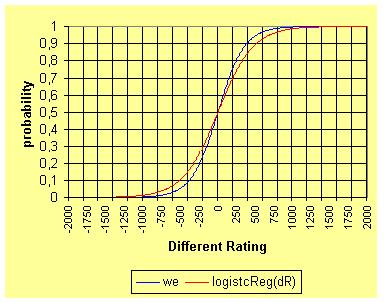ELO Rating The Elo rating System is used since 1970 by the Chess federation to rank their players. The System was created by the mathematician and chess player Arpad Elo. It can be used for sport ranking too, with the advantage that the differences in rating can be converted into scoring or winning probabilities witch can be compare with the odds form the bookmaker. Conversely, scoring percentages can be converted into differences in ratings. The player with the higher ranking has a higher probability (win expectancy) to win the match. The probability that Player A would defeat Player B is is calculated by the formula: p = win expectancy = 1/(1+10^[(Rb-Ra)/400])Ra is Player A's rating (team A) and Rb is the rating for Player B (team B) before the match. If for example Andre Agassi with rating 2207.4 plays his next matches against Wayne Ferreira with ranking 1991.9. The Ranking difference is 1991.9-2207.4=-215.5. Then the win expectancy for Agassi is 1/(1+10^[(1991.9 -2207.4)/400])= 0,776 and for Ferreira = 1-0,776=0,224. That means fair (European) odds are 1/0.776=1.29 for Agassi and 1/0.224=4.46 Ferreira. All bets with higher odds are promising profitable assuming the Elo win expectancy is accurate. With the win expectancy from the Elo-ranking we can identify profitable bets. The advantage over for the bookmaker's, is calculated by multiplying the win expectancy from Eloranking with the odds from the bookmaker minus one. Expected profit (advantage) = win expectancy*odds-1 If the calculated advantage is higher then zero the bet can be profitable (odds European) (see calculator). The rating for each player can be calculated with the following steps: 1. Assigned initial ratings, between approximately 1000 and 2000 to all players (for all Players the same). 2. Each player's rating will be adjusted after each game by a factor, calculated using the formula : ``` 1 Ra_new = Ra_old + K (w - -----------------) (Rb-Ra)/400 1 + 10 ``` Suppose Player A rating is Ra, and the opponent's is Rb. Let w be 1 if Player A win, 0.5 if draw, and 0 if Player A lose. After the game, Players A new rating will be Ra_new. K = 32 (for Chess rating, speer of convergence) 3. This has to be done for every match. When this cycle is finished, a rating for each player is obtained. These ratings are used as initial ratings for the next round of calculation. The process repeats iteratively until the ratings remain stable without further change. For more Information on ranking system see the links below. Home